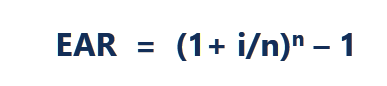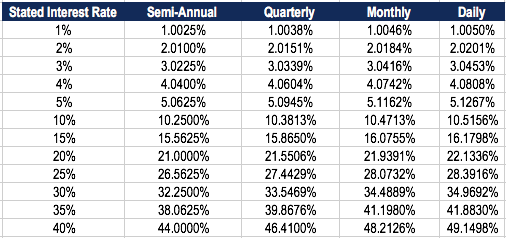# Effective Annual Interest Rate

Interest rate adjusted for compounding over a given period

## What is the Effective Annual Interest Rate?

The Effective Annual Rate (EAR) is the interest rate that is adjusted for compounding over a given period. Simply put, the effective annual interest rate is the rate of interest that an investor can earn (or pay) in a year after taking into consideration compounding.

The Effective Annual Interest Rate is also known as the effective interest rate, effective rate, or the annual equivalent rate. Compare it to the Annual Percentage Rate (APR) which is based on simple interest.

The EAR formula for Effective Annual Interest Rate:Where:

i = stated annual interest rate

n = number of compounding periods

### Effective Annual Rate Based on Compounding

The table below shows the difference in the effective annual rate when the compounding periods change.Table: CFI’s Free Fixed Income Fundamentals Course.

For example, the EAR of a 1% Stated Interest Rate compounded quarterly is 1.0038%.

### Importance of Effective Annual Rate

The Effective Annual Interest Rate is an important tool that allows the evaluation of the true return on an investment or true interest rate on a loan.

The stated annual interest rate and the effective interest rate can be significantly different, due to compounding. The effective interest rate is important in figuring out the best loan or determining which investment offers the highest rate of return.

In the case of compounding, the EAR is always higher than the stated annual interest rate.

### Example of Effective Interest Rate

For example, assume the bank offers your deposit of \$10,000 a 12% stated interest rate compounded monthly. The table below demonstrates the concept of the effective annual interest rate:Table: CFI’s Free Fixed Income Fundamentals Course.

Month 1 Interest: Beginning Balance (\$10,000) x Interest Rate (12%/12 = 1%) = \$100

Month 2 Interest: Beginning Balance (\$10,100) x Interest Rate (12%/12 = 1%) = \$101

The change, in percentage, from the beginning balance (\$10,000) to the ending balance (\$11,268) is (\$11,268 – \$10,000)/\$10,000 = .12683 or 12.683%, which is the effective annual interest rate. Even though the bank offered a 12% stated interest rate, your money grew by 12.683% due to monthly compounding.

The effective annual interest rate allows you to determine the true return on investment (ROI).

Enter your name and email in the form below and download the free template shown above now!

### How to Calculate the Effective Interest Rate?

To calculate the effective interest rate using the EAR formula, follow these steps:

#### 1. Determine the stated interest rate

The stated interest rate (also called annual percentage rate or nominal rate) is usually found in the headlines of the loan or deposit agreement. Example: “Annual rate 36%, interest charged monthly.”

#### 2. Determine the number of compounding periods

The compounding periods are typically monthly or quarterly. The monthly compounding periods may be 12 (12 months in a year) and 4 for quarterly (4 quarters in a year).

• Monthly = 12 compounding periods
• Quarterly = 4 compounding periods
• Bi-Weekly = 26 compounding periods
• Weekly = 52 compounding periods
• Daily = 365 compounding periods

#### 3. Apply the EAR Formula: EAR = (1+ i/n)n – 1

Where:

i = stated interest rate

n = compounding periods

### Example

To calculate the effective annual interest rate of a credit card with an annual rate of 36% and interest charged monthly:

1. Stated interest rate: 36%

2. Number of compounding periods: 12

Therefore, EAR = (1+0.36/12)^12 – 1 = 0.4257 or 42.57%.

### Why Don’t Banks Use The Effective Annual Interest Rate?

When banks are charging interest, the stated interest rate is used instead of the effective annual interest rate. This is done to make consumers believe that they are paying a lower interest rate.

For example, for a loan at a stated interest rate of 30%, compounded monthly, the effective annual interest rate would be 34.48%. Banks will typically advertise the stated interest rate of 30% rather than the effective interest rate of 34.48%.

When banks are paying interest on your deposit account, the effective annual rate is advertised to look more attractive than the stated interest rate.

For example, for a deposit at a stated rate of 10% compounded monthly, the effective annual interest rate would be 10.47%. Banks will advertise the effective annual interest rate of 10.47% rather than the stated interest rate of 10%.

Essentially, they show whichever rate appears more favorable.

Thank you for reading this guide to understand the Effective Annual Rate formula. CFI is a global provider of financial modeling courses and financial analyst certification. To continue developing your career as a financial professional, check out the following additional resources:

• Expected Return
• Basis Points
• Capital Gains Yield
• Weighted Average Cost of Capital (WACC)

### Financial Analyst Training

Get world-class financial training with CFI’s online certified financial analyst training program!

Gain the confidence you need to move up the ladder in a high powered corporate finance career path.

Learn financial modeling and valuation in Excel the easy way, with step-by-step training.